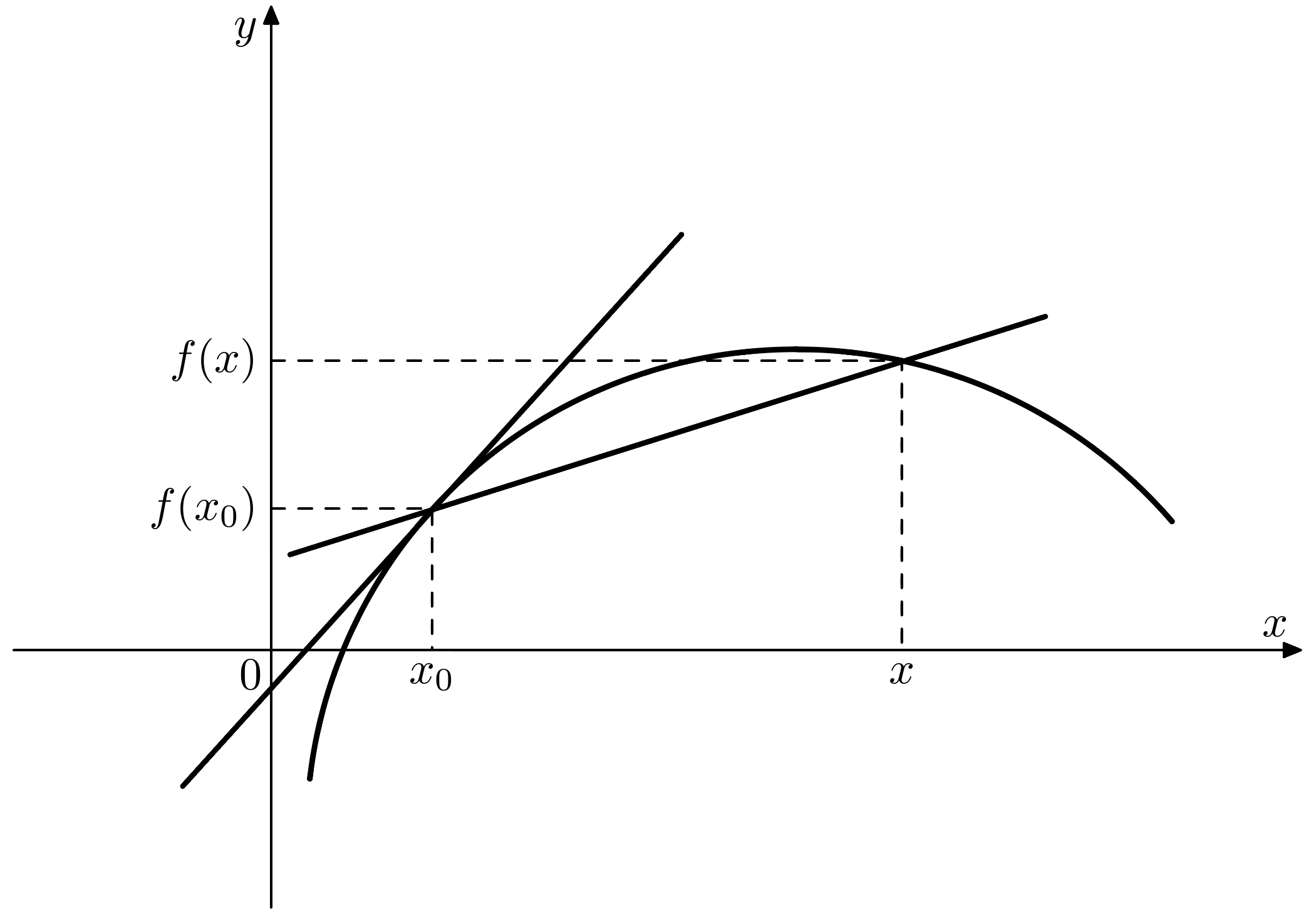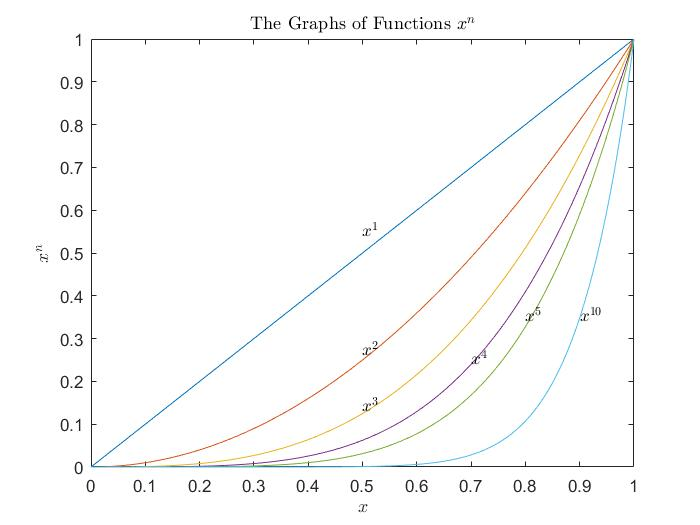# 导数定义与性质

[定义][导数][定义][微分]

[定理][有限增量公式]

$$\Delta y= f(x)-f(x_0)= f’(x_0)\Delta x +o(\Delta),\quad x\rightarrow x_0.$$

$$\Delta y = f’(x_0)\Delta x+\omega(x)\Delta x$$

[定理]

- $(f+g)’(x)=f’(x)+g’(x)$;
- $(fg)’(x)=f’(x)g(x)+f(x)g’(x)$;
- 若$g(x)\neq 0$, $\left(\frac{f}{g}\right)’ (x) = \frac{g(x)f’(x)-g’(x)f(x)}{g^2(x)}$.

[定理][链式法则]

[定义][单调]

[定理][反函数求导法则]

$$\varphi’(y)=\frac{1}{f’(x)}=\frac{1}{f’(\varphi(y))}.$$

[定义]

$$f,f’’,f^{(3)},\cdots,f^{(n)}$$

\begin{array}{c}
(uv)^{(n)} & = & C_{n}^{0}u^{0}v^{n}+C_{n}^{1}u’v^{(n-1)}+C_{n}^{2}u’’v^{(n-2)}+\cdots+C_{n}^{n}u^{(n)}v^{(0)}\\
\end{array}[举例]

$$f(x):=\begin{cases} e^{-\frac{1}{x^2}},\quad x\neq 0, \ 0,\quad x=0, \end{cases}.$$

# 可微函数性质

## 中值定理

[定义]

[定理][Taylor]

[定理][Cauchy 中值定理]

$$[g(b)-g(a)]f’(\xi)=[f(b)-f(a)]g’(\xi).$$

[定理][Lagrange 中值定理]

$$\frac{f(b)-f(a)}{b-a}=f’(\xi).$$

[定理][Rolle 中值定理]

$$f’(\xi)=0.$$

[定理][达布(Darboux)定理]

[定理][导函数极限定理]

[定理][洛必达(L’Hospital)法则]

$$\lim_{x\rightarrow a+}f(x)=\lim_{x\rightarrow a+}g(x)=0,$$

$$\lim_{x\rightarrow a+}g(x)=\infty,$$

$$\lim_{x\rightarrow a+}\frac{f’(x)}{g’(x)}=A$$

$$\lim_{x\rightarrow a+}\frac{f(x)}{g(x)}=\lim_{x\rightarrow a+}\frac{f’(x)}{g’(x)}=A.$$

[引理1]

$$\mu(x_2-x_1)\leqslant f(x_2)-f(x_1)\leqslant\eta(x_2-x_1).$$

[引理2]

[定理]

$$f’(x_0)=0, f’’(x_0)<(>)0$$

[引理3]

## 泰勒公式

[定理][(带Peano余项的)Taylor 公式]

$$f(x)= \sum_{k=0}^{n}\frac{1}{k!}f^{(k)}(x_0)(x-x_0)^k +o((x-x_0)^n), \quad (x\rightarrow x_0).$$

[定理][Taylor 中值定理]

$$f(x)= \sum_{k=0}^{n}\frac{1}{k!}f^{(k)}(x_0)(x-x_0)^k + \frac{1}{(n+1)!}f^{(n+1)}(\xi)(x-x_0)^{n+1}$$

$$f(x)= \sum_{k=0}^{n}\frac{1}{k!}f^{(k)}(x_0)(x-x_0)^k + \frac{1}{n!}f^{(n+1)}(\zeta)(x-\zeta)^n(x-x_0).$$

# 应用:关于微分方程的一些结论

$$y’=\phi(x,y),\quad y(a)=c$$

[定理]

\begin{proof}

[引理]

\begin{array}{c}
|F(x_1)|=|F(x_1)-F(x_0)|\leqslant |x_1-x_0|\sup_{\xi\in I}|F’(x)|\\
\leqslant A\sup_{\xi\in I}|F(x)||x_1-x_0|\leqslant A\delta|F(x_1)|=\frac{1}{2}|F(x_1)|.
\end{array}

$$F(a)=0.$$

$$|F’(x)|=|\phi(x,f_1(x))-\phi(x,f_2(x))|\leqslant A|f_1(x)-f_2(x)|.$$

\end{proof}

$$f’(x)\leqslant \gamma f(x)^\beta$$

$$g’(x)=(1-\beta) f^{-\beta}(x)f’(x)\leqslant \gamma(1-\beta).$$

$$g(x)\leqslant\gamma(1-\beta)(x-a)+c.$$

• $$f’=\gamma f.$$这种情况,$f(x)$正比于函数$e^{\gamma x}$, 也就是说这个解函数成指数级增长(exponential growth);
• $$f’=\gamma f^{\alpha}\quad(\alpha>1).$$这种情况下, 正的解函数呈现爆炸式的增长,也就是说在某些有限的区间上,函数值可以向无穷大增长.有时也称其双曲增长(Hyperbolic growth).
• $$f’=\gamma f^{\beta}\quad(\beta<1).$$这种情况下, 解函数最多呈多项式增长(Polynominal growth).
• 当然,最简单的情况就是$f’=\gamma$.此时, 解函数呈线性增长(Linear growth).

Zengfk

2018-01-22

2019-08-04